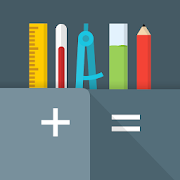All-In-One CalculatorEveryone
112,273
·
Offers in-app purchasesEnjoy this app for free, plus many more free of ads and in-app purchases, with a Google Play Pass subscription. Try free for 1 month.
The original All-In-One Calculator for Android
It's a FREE, complete and easy to use multi calculator & converter.

What does it do?
Designed with simplicity in mind, it helps you solve everyday problems.
From simple or complex calculations, to unit and currency conversions, percentages, proportions, areas, volumes, etc... it does it all. And it does it good!

This is the PERFECT Calculator
Passionate development combined with constant feedback we receive from our users resulted in that we think is the best multi calculator on the store.
Featuring over 75 FREE Calculators and Unit Converters packed in with a Simple or Scientific Calculator, it's the only calculator you will need from now on on your device.

Oh, and did we say it is completely FREE?
Yes, it is free. We think everyone should enjoy this.

If you are a student, teacher, engineer, handyman, contractor or just somebody who struggles with math & conversions, you really should give this one a try.
• Use it for simple or complex calculations
• Convert units or currencies in the same app
• Enjoy easier homework or school assignments
• Learn. See step by step solutions to your calculations

So, on with the features...

MAIN CALCULATOR
• Simple or Scientific layout
• Editable input & cursor
• Copy & Paste support
• Calculation history
• Memory buttons
• Function graphing
• Dec, Hex & Binary
• Floating calculator
• Widget

75 CALCULATORS & CONVERTERS
• Algebra, Geometry, Unit Converters, Finance, Health & Misc
• Currency converter with 160 currencies (available offline)
• Instant results delivered as you type
• Step-by-step solution & formulas
• Smart search for faster navigation
• Create shortcuts on home screen

Algebra
• Percentage calculator
• Proportion calculator
• Ratio calculator
• Average calculator - arithmetic, geometric and harmonic means
• Equation solver - linear, quadratic and equation system
• Combinations and permutations
• Decimal to fraction
• Fraction simplifier
• Prime number checker
• Greatest common factor & Lowest common multiple calculator
• Random number generator

Geometry
• Shape calculators for square, rectangle, parallelogram, trapezoid, rhombus, triangle, pentagon, hexagon, circle, circle arc, ellipse
• Body calculators for cube, rect. prism, square pyramid, sq. pyramid frustum, cylinder, cone, conical frustum, sphere, spherical cap, spherical frustum, ellipsoid

Unit converters
• Acceleration converter
• Angle converter
• Length converter
• Energy converter
• Force converter
• Torque converter
• Area converter
• Volume converter
• Volumetric flow converter
• Weight converter
• Temperature converter
• Pressure converter
• Power converter
• Speed converter
• Mileage converter
• Time converter
• Digital storage converter
• Data transfer speed converter
• Numeric base converter
• Roman numerals converter
• Shoe size converter
• Ring size converter
• Cooking converter

Finance
• Currency converter with 160 currencies available offline
• Unit price calculator
• Sales tax calculator
• Tip calculator
• Loan calculator
• Simple / Compound interest calculator

Health
• Body mass index - BMI
• Daily calories burn
• Body fat percentage

Miscellaneous
• Age calculator
• Date calculator
• Time calculator
• Mileage calculator
• Ohm's law calculator - voltage, current, resistance and power

Developed in Transylvania 🇷🇴
Collapse

Reviews

Review policy and info
4.7
112,273 total
5
4
3
2
1

What's New

Version 2.2.1
• Added Azerbaijani, Bulgarian, Chinese (traditional), Finnish, Hebrew, Persian and Turkish translations.

Join the translation effort here: bit.ly/2xzUcbm
Collapse

Updated
January 10, 2022
Size
11M
Installs
5,000,000+
Current Version
2.2.1
Requires Android
5.0 and up
Content Rating
Everyone
In-app Products
\$4.99 per item
Permissions
Offered By
allinonecalculator.com
Developer
Prof. Nicolae Margineanu 22B, Cluj Napoca, Romania.